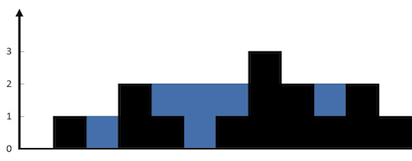## Trapping Rain Water

06/26/2016 Array Stack Two Pointers

## Question

Given n non-negative integers representing an elevation map where the width of each bar is 1, compute how much water it is able to trap after raining.

For example,

Given [0,1,0,2,1,0,1,3,2,1,2,1], return 6.The above elevation map is represented by array [0,1,0,2,1,0,1,3,2,1,2,1]. In this case, 6 units of rain water (blue section) are being trapped.

Thanks Marcos for contributing this image!

## Solution

Result: Accepted Time: 4ms

Here should be some explanations.

int trap(int* height, int hsz) {
int left = 0, right = hsz - 1,ret = 0,hlast = 0;
while(left < right)
{
int tmp = (height[left] < height[right])?height[left++]:height[right--];
if(tmp > hlast)
hlast = tmp;
else
ret += hlast - tmp;
}
return ret;
}


Complexity Analytics

• Time Complexity: $O(n)$
• Space Complexity: $O(1)$